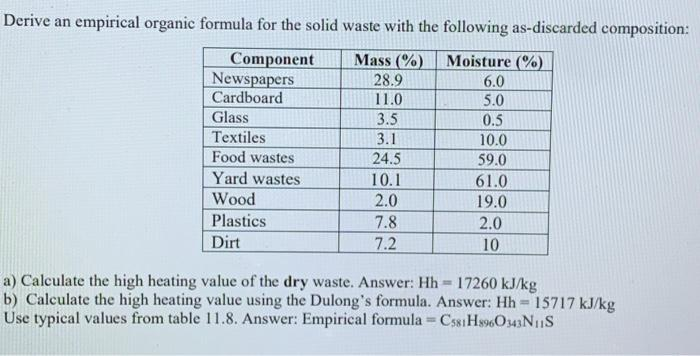# (Solved): Derive an empirical organic formula for the solid waste with the following as-discarded composition ...Derive an empirical organic formula for the solid waste with the following as-discarded composition: a) Calculate the high heating value of the dry waste. Answer: b) Calculate the high heating value using the Dulong's formula. Answer: Use typical values from table 11.8. Answer: Empirical formula

We have an Answer from Expert

The empirical organic formula for the solid waste is   based on typical elemental compositions and assumptions.

calculate the approximate mass of each element in the solid waste based on the given mass percentages:

Moisture:
Mass:
=
We have an Answer from Expert